# Understanding TL431 Internal Architecture - Help needed

#### mishra87

Joined Jan 17, 2016
864
Hi Guys,

I am trying to understand TL431 internal architecture working but i did not understand completely.
Could anyone help me out here to make me understand how TL431 works ?
I do not want to understand the how do we calculate Vout. I just wanted to Understand this internal opamp and transistor works to regulate the output.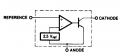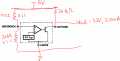#### mishra87

Joined Jan 17, 2016
864
You have the TL431 connected wrong. For the correct way, refer to Fig. 27 in the data sheet:

View attachment 214805
As for how the TL431 works, the data sheet gives a good description in Section 10.2.2, beginning on page 25.
I figured out my mistakes and corrected the drawing.
description in Section 10.2.2, beginning on page 25 :
I know how to design and bias the TL431 as many datasheet explained very well. My area of intreset here is to learn how opamp and transistor works for desired output voltage.

Let me try to explain what i wanted to understand here!

Case1 : Lets say Vout = 3.3V. The opamp works as a positive comparator so whenever output goes above 3.3V voltage at V+ of goes up and opamp output goes towards positive saturation and above 2.5V(V-) and that drive transistor base. I am not sure if transistor working in linear region or saturation to maintain the output constant.

Case 2: Whenever output goes below 3.3V. The voltage at reference(V+) pin goes below to 2.5V (V-) and opamp goes towards negative saturation and transistor will off and i am not sure what is the output now.

I just wanted to understand my assumption to understand the working of TL431 internal block.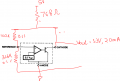#### crutschow

Joined Mar 14, 2008
25,994
The opamp operation can be readily visualized by noting that it, and the transistor, are always operating in the linear feedback mode for normal voltage regulator operation, and thus will always try to keep the differential voltage between its plus an minus inputs very near zero (as determined by the opamp high open-loop gain).

So when the output from the voltage divider at the VREF input tries to go above the Vref voltage , the opamp increases the voltage (current) to the transistor base to turn it further on (draw more current) and pull the voltage back down.

Similarly, if the output tries to go lower than the Vref voltage, the opamp reduces the voltage (current) to the transistor base to reduce its current, causing the voltage to rise.

Last edited:

#### ronsimpson

Joined Oct 7, 2019
1,122

#### OBW0549

Joined Mar 2, 2015
3,566
@crutschow explains it about as neatly as it can be explained.

I am not sure if transistor working in linear region or saturation to maintain the output constant.
Ordinary common sense should tell you that it is working in the linear region; the TL431 wouldn't be very useful otherwise, would it?

Case 2: Whenever output goes below 3.3V. The voltage at reference(V+) pin goes below to 2.5V (V-) and opamp goes towards negative saturation and transistor will off and i am not sure what is the output now.
You need to learn to distinguish between situations where something is functioning as a switch (+/- saturation, ON/OFF, etc.) and situations where it is functioning in a linear fashion. Usually, common sense should be your guide and there's no way any of us can teach you that.

Try harder.

#### mishra87

Joined Jan 17, 2016
864

#### mishra87

Joined Jan 17, 2016
864
@crutschow explains it about as neatly as it can be explained.

Ordinary common sense should tell you that it is working in the linear region; the TL431 wouldn't be very useful otherwise, would it?

You need to learn to distinguish between situations where something is functioning as a switch (+/- saturation, ON/OFF, etc.) and situations where it is functioning in a linear fashion. Usually, common sense should be your guide and there's no way any of us can teach you that.

Try harder.

#### mishra87

Joined Jan 17, 2016
864
The opamp operation can be readily visualized by noting that it, and the transistor, are always operating in the linear feedback mode for normal voltage regulator operation, and thus will always try to keep the differential voltage between its plus an minus inputs very near zero (as determined by the opamp high open-loop gain).

So when the output from the voltage divider at the VREF input tries to go above the Vref voltage , the opamp increases the voltage (current) to the transistor base to turn it further on (draw more current) and pull the voltage back down.

Similarly, if the output tries to go lower than the Vref voltage, the opamp reduces the voltage (current) to the transistor base to reduce its current, causing the voltage to rise.

That is what i wanted to understand and learn !
So now i know transistor is working in linear region. I still have few doubts if you explain me a bit to conclude it.

1. You say, opamp works in linear feedback mode :
i see transistor collector is connected to opamp V+ so how linear feedback mode works for TL431 ?

2. If opamp Vo increases more base current the Vce of transistor decreases so i assume that voltage drop will be at series resistor 76.8ohm. please correct me if i am wrong ?

3. If opamp Vo decreases more base current the Vce of transistor increases so i assume that voltage drop will be at Vce of transistor. please correct me if i am wrong ?

Thanks once again !

#### OBW0549

Joined Mar 2, 2015
3,566
i see transistor collector is connected to opamp V+ so how linear feedback mode works for TL431 ?
You're failing to consider the inversion due to the transistor.

In situations like this, to determine whether feedback is positive or negative, it helps to "walk around the feedback loop" while applying an imaginary disturbance at some point (here, the op amp's non-inverting input):

1) An increase in voltage at the op amp's non-inverting input causes the op amp's output to move positive;
2) The increased op amp output voltage causes the transistor to turn on more;
3) The transistor's increased collector current pulls down the V+ line;
4) The decrease in V+ causes the voltage at the op amp's non-inverting input to decrease, counteracting the original disturbance we introduced in step 1.

Therefore, the feedback is negative.

#### mishra87

Joined Jan 17, 2016
864
You're failing to consider the inversion due to the transistor.

In situations like this, to determine whether feedback is positive or negative, it helps to "walk around the feedback loop" while applying an imaginary disturbance at some point (here, the op amp's non-inverting input):

1) An increase in voltage at the op amp's non-inverting input causes the op amp's output to move positive;
2) The increased op amp output voltage causes the transistor to turn on more;
3) The transistor's increased collector current pulls down the V+ line;
4) The decrease in V+ causes the voltage at the op amp's non-inverting input to decrease, counteracting the original disturbance we introduced in step 1.

Therefore, the feedback is negative.
Thank you very much for being such a mentor and teaching me like beginner.

Last edited:

#### Marley

Joined Apr 4, 2016
384

By Ken Sherriff. From his blog - always full of interesting stuff.

•OBW0549 and ci139

#### ci139

Joined Jul 11, 2016
1,696
XX431 appears actually a quite state of the art shunting voltage regulator -- it's because of it's "stability"/transient-response
(if you copy any of the following directly into the Spice simulation -- they won't work ... even near correct ! -- see article in the prev post #12 ... )
(( also your simplified Op-Amp equivalent likely won't ... with few tricks it can be made "better" ... that is - "a better-match" ))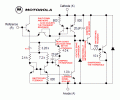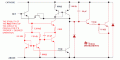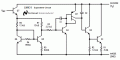Last edited:

#### ronsimpson

Joined Oct 7, 2019
1,122
Spice simulation -- they won't work ... even near correct
I think SPICE needs to know that the transistors are not all the same. The size of the transistors (emitters) is different. Part of the key is the ratio of resistors and the ratio of transistor sizes. In a simple SPICE file using the same transistors you miss the real type of transistors used.

By now all can see, every drawing of the inside of the TL431 is different.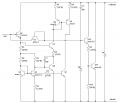It took too long for me to understand the bottom two transistors make a comparitor. If the voltage or current at the two inputs are equal then the collector current in the bottom two transistors are equal. The top transistor pair will see equal currents and the voltage at "20pF" will not change. If there is any imbalance then the output will move up or down ward.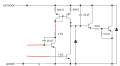#### ci139

Joined Jul 11, 2016
1,696
plot the Ic (for the one with the 1kΩ on it's base) for a varying input (cathode) current so the I.shunt approaches the I.max for the '431 -- it'll be quite interesting